# Minkowski diagram

﻿
Minkowski diagram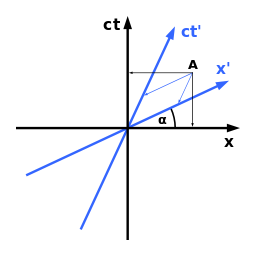In the theory of relativity each observer assigns the event at A to a different time and location.

The Minkowski diagram was developed in 1908 by Hermann Minkowski and provides an illustration of the properties of space and time in the special theory of relativity. It allows a quantitative understanding of the corresponding phenomena like time dilation and length contraction without mathematical equations.

The term Minkowski diagram is used in both a generic and particular sense. In general, a Minkowski diagram is a graphic depiction of a portion of Minkowski space, often where space has been curtailed to a single dimension. These two-dimensional diagrams portray worldlines as curves in a plane that correspond to motion along the spatial axis. The vertical axis is usually temporal, and the units of measurement are taken such that the light cone at an event consists of the lines of slope plus or minus one through that event.

A particular Minkowski diagram illustrates the result of a Lorentz transformation. The origin corresponds to an event where a change of velocity takes place. The new worldline forms an angle α with the vertical, with α < π/4. The Lorentz transformation that moves the vertical to α also moves the horizontal by α. The horizontal corresponds to the usual notion of simultaneous events, for a stationary observer at the origin. After the Lorentz transformation the new simultaneous events lie on the α-inclined line. Whatever the magnitude of α, the line t = x forms the universal bisector.

In Minkowski’s 1908 paper there were three diagrams, first to illustrate the Lorentz transformation, then the partition of the plane by the light-cone, and finally illustration of worldlines. The first diagram used a branch of the unit hyperbola t2x2 = 1 to show the locus of a unit of proper time depending on velocity, thus illustrating time dilation. The second diagram showed the conjugate hyperbola to calibrate space, where a similar stretching leaves the impression of Fitzgerald contraction. In 1914 Ludwik Silberstein included a diagram of "Minkowski’s representation of the Lorentz transformation". This diagram included the unit hyperbola, its conjugate, and a pair of conjugate diameters. Since the 1960s a version of this more complete configuration has been referred to as The Minkowski Diagram, and used as a standard illustration of the transformation geometry of special relativity. E. T. Whittaker has pointed out that the Principle of relativity is tantamount to the arbitrariness of what hyperbola radius is selected for time in the Minkowski diagram. In 1912 Gilbert N. Lewis and Edwin B. Wilson applied the methods of synthetic geometry to develop the properties of the non-Euclidean plane that has Minkowski diagrams.

## BasicsChoosing ct instead of t on the time axis the world line of a photon becomes a straight line with a slope of 45°.

For simplification in Minkowski diagrams, usually only events in a one dimensional world are considered. Unlike common distance-time diagrams, the distance will be displayed on the x-axis (abscissa) and the time on the y-axis (ordinate). In this manner the events happening on a horizontal path in reality can be transferred easily to a horizontal line in the diagram. Objects plotted on the diagram can be thought of as moving from bottom to top as time passes. In this way each object, like an observer or a vehicle, follows in the diagram a certain curve which is called its world line.

Each point in the diagram represents a certain position in space and time. Such a position is called an event whether or not anything happens at that position.

For convenience, the (vertical) time axis represents, not t, but the corresponding quantity ct, where c =299,792,458 m/s is the speed of light. In this way, one second on the ordinate corresponds to a distance of 299,792,458 m on the abscissa. Due to x=ct for a photon passing through the origin to the right, its world line is a straight line with a slope of 45°, if the scales on both axes are chosen to be identical.

## Path-time diagram in Newtonian physics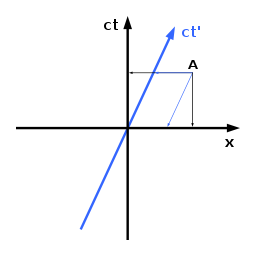In Newtonian physics for both observers the event at A is assigned to the same point in time.

The adjoining diagram shows the coordinate system of an observer which we will refer to as 'at rest', and who is positioned at x=0. His world line is identical with the time axis. Each parallel line to this axis would correspond also to an object at rest but at another position. The blue line, however, describes an object moving with constant speed v to the right, such as a moving observer.

This blue line can be interpreted as the time axis for the second observer. Together with the path axis (which is identical for both observers) it represents his coordinate system. This corresponds with the agreement between both observers to denote the position x=0 and t=0 also with x'=0 and t'=0. The axes for the moving observer are not perpendicular to each other and the scale on his time axis is stretched. To determine the coordinates of a certain event, two lines parallel to the two axes must be constructed passing through the event, and their intersections with the axes read off.

Determining position and time of the event A as an example in the diagram leads to the same time for both observers, as expected. Only for the position different values result, because the moving observer has approached the position of the event A since t=0. Generally stated, all events on a line parallel to the path axis happen simultaneously for both observers. There is only one universal time t=t' which corresponds with the existence of only one common path axis. On the other hand due to two different time axes the observers usually measure different path coordinates for the same event. This graphical translation from x and t to x' and t' and vice versa is described mathematically by the so called Galilean transformation.

## Minkowski diagram in special relativityIn the theory of relativity both observers assign the event at A to different times and locations.

Albert Einstein discovered that the description above is not correct. Space and time have properties which lead to different rules for the translation of coordinates in case of moving observers. In particular, events which are estimated to happen simultaneously from the viewpoint of one observer, happen at different times for the other.

In the Minkowski diagram this relativity of simultaneity corresponds with the introduction of a separate path axis for the moving observer. Following the rule described above each observer interprets all events on a line parallel to his path axis as simultaneous. The sequence of events from the viewpoint of an observer can be illustrated graphically by shifting this line in the diagram from bottom to top.

If ct instead of t is assigned on the time axes, the angle α between both path axes will be identical with that between both time axes. This follows from the second postulate of the special relativity, saying that the speed of light is the same for all observers, regardless of their relative motion (see below). α is given by$\tan(\alpha) = \frac{v}{c}$.

The corresponding translation from x and t to x' and t' and vice versa is described mathematically by the so called Lorentz transformation. Whatever space and time axes arise through such transformation, in a Minkowski diagram they correspond to conjugate diameters of a pair of hyperbolas.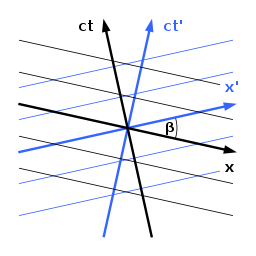Symmetrical representation with lines of simultaneity for both observers.

For the graphical translation it has been taken into account that the scales on the inclined axes are different from the Newtonian case described above. To avoid this problem it is recommended that the whole diagram be deformed in such a way that the scales become identical for all axes, eliminating any need to stretch or compress either axis. This can be done by a compression in the direction of 45° or an expansion in the direction of 135° until the angle between the time axes becomes equal to the angle between the path axes. The angle β between both time and path axes is given by$\sin(\beta) = \frac{v}{c}$.

In this symmetrical representation (also referred to as Loedel diagram, named after the physicist Enrique Loedel Palumbo who first introduced this symmetrised Minkowski representation), the coordinate systems of both observers are equivalent, since both observers are traveling at the same speed in opposite directions, relative to some third point of view.

However, Loedel diagrams become more complicated than Minkowski diagrams for more than three observers and therefore lose their pedagogical appeal.

## Time dilation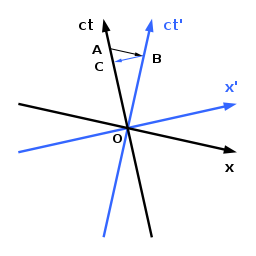Time dilation: Both observers consider the clock of the other as running slower.

Relativistic time dilation means that a clock moving relative to an observer is running slower and finally also the time itself in this system. This can be read immediately from the adjoining Minkowski diagram. The observer at A is assumed to move from the origin O towards A and the clock from O to B. For this observer at A all events happening simultaneously in this moment are located on a straight line parallel to its path axis passing A and B. Due to OB<OA he concludes that the time passed on the clock moving relative to him is smaller than that passed on his own clock since they were together at O.

A second observer having moved together with the clock from O to B will argue that the other clock has reached only C until this moment and therefore this clock runs slower. The reason for these apparently paradoxical statements is the different determination of the events happening synchronously at different locations. Due to the principle of relativity the question of "who is right" has no answer and does not make sense.

## Length contraction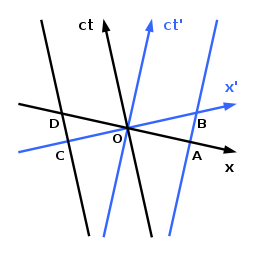Length contraction: Both observers consider objects moving with the other observer as being shorter.

Relativistic length contraction means that the length of an object moving relative to an observer is decreased and finally also the space itself is contracted in this system. The observer is assumed again to move along the ct-axis. The world lines of the endpoints of an object moving relative to him are assumed to move along the ct'-axis and the parallel line passing through A and B. For this observer the endpoints of the object at t=0 are O and A. For a second observer moving together with the object, so that for him the object is at rest, it has the length OB at t'=0. Due to OA<OB the object is contracted for the first observer.

The second observer will argue that the first observer has evaluated the endpoints of the object at O and A respectively and therefore at different times, leading to a wrong result due to his motion in the meantime. If the second observer investigates the length of another object with endpoints moving along the ct-axis and a parallel line passing through C and D he concludes the same way this object to be contracted from OD to OC. Each observer estimates objects moving with the other observer to be contracted. This apparently paradoxical situation is again a consequence of the relativity of simultaneity as demonstrated by the analysis via Minkowski diagram.

For all these considerations it was assumed, that both observers take into account the speed of light and their distance to all events they see in order to determine the times at which these events happen actually from their point of view.

## Constancy of the speed of light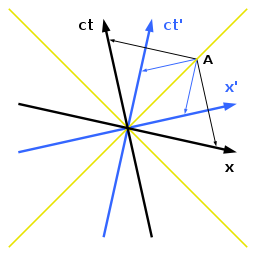For the speed of a photon passing A both observers measure the same value even though they move relative to each other.

Another postulate of special relativity is the constancy of the speed of light. It says that any observer in an inertial reference frame measuring the speed of light relative to himself obtains the same value regardless of his own motion and that of the light source. This statement seems to be paradox, but it follows immediately from the differential equation yielding this, and the Minkowski diagram agrees. It explains also the result of the Michelson–Morley experiment which was considered to be a mystery before the theory of relativity was discovered, when photons were thought to be waves through an undetectable medium.

For world lines of photons passing the origin in different directions x=ct and x=−ct holds. That means any position on such a world line corresponds with steps on x- and ct-axis of equal absolute value. From the rule for reading off coordinates in coordinate system with tilted axes follows that the two world lines are the angle bisectors of the x- and ct-axis. The Minkowski diagram shows, that they are angle bisectors of the x'- and ct'-axis as well. That means both observers measure the same speed c for both photons.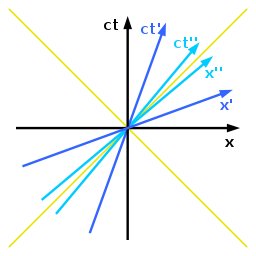Minkowski diagram for 3 coordinate systems. For the speeds relative to the system in black v' = 0.4c and v" = 0.8c holds.

In principle further coordinate systems corresponding to observers with arbitrary velocities can be added in this Minkowski diagram. For all these systems both photon world lines represent the angle bisectors of the axes. The more the relative speed approaches the speed of light the more the axes approach the corresponding angle bisector. The path axis is always more flat and the time axis more steep than the photon world lines. The scales on both axes are always identical, but usually different from those of the other coordinate systems.

## Speed of light and causality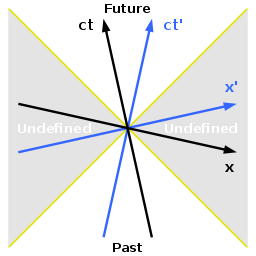Past and future relative to the origin. For the grey areas a corresponding temporal classification is not possible.

Straight lines passing the origin which are steeper than both photon world lines correspond with objects moving more slowly than the speed of light. If this applies to an object, then it applies from the viewpoint of all observers, because the world lines of these photons are the angle bisectors for any inertial reference frame. Therefore any point above the origin and between the world lines of both photons can be reached with a speed smaller than that of the light and can have a cause-effect-relationship with the origin. This area is the absolute future, because any event there happens later compared to the event represented by the origin regardless of the observer, which is obvious graphically from the Minkowski diagram.

Following the same argument the range below the origin and between the photon world lines is the absolute past relative to the origin. Any event there belongs definitely to the past and can be the cause of an effect at the origin.

The relationship between of such pairs of event is called timelike, because they have a finite time distance different from zero for all observers. On the other hand a straight line connecting these two events is always the time axis of a possible observer for whom they happen at the same place. Two events which can be connected just with the speed of light are called lightlike.

In principle a further dimension of space can be added to the Minkowski diagram leading to a three-dimensional representation. In this case the ranges of future and past become cones with apexes touching each other at the origin. They are called light cones.

## The speed of light as a limit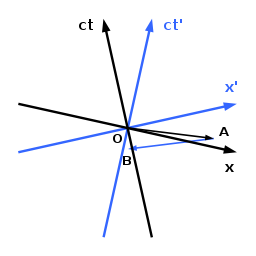Sending a message at superluminal speed from O via A to B into the past. Both observers consider the temporal order of the pairs of events O and A as well as A and B different.

Following the same argument, all straight lines passing through the origin and which are more nearly horizontal than the photon world lines, would correspond to objects or signals moving faster than light regardless of the speed of the observer. Therefore no event outside the light cones can be reached from the origin, even by a light-signal, nor by any object or signal moving with less than the speed of light. Such pairs of events are called spacelike because they have a finite spatial distance different from zero for all observers. On the other hand a straight line connecting such events is always the space coordinate axis of a possible observer for whom they happen at the same time. By a slight variation of the velocity of this coordinate system in both directions it is always possible to find two inertial reference frames whose observers estimate the chronological order of these events to be different.

Therefore an object moving faster than light, say from O to A in the adjoining diagram, would imply that, for any observer watching the object moving from O to A, there can be found another observer (moving at less than the speed of light with respect to the first) for whom the object moves from A to O. The question of which observer is right has no unique answer, and therefore makes no physical sense. Any such moving object or signal would violate the principle of causality.

Also, any general technical means of sending signals faster than light would permit information to be sent into the originator's own past. In the diagram, an observer at O in the x-ct-system sends a message moving faster than light to A. At A it is received by another observer, moving so as to be in the x'-ct'-system, who sends it back, again faster than light by the same technology, arriving at B. But B is in the past relative to O. The absurdity of this process becomes obvious when both observers subsequently confirm that they received no message at all but all messages were directed towards the other observer as can be seen graphically in the Minkowski diagram. Indeed, if it was possible to accelerate an observer to the speed of light, the space and time axes would coincide with their angle bisector. The coordinate system would collapse.

These considerations show that the speed of light as a limit is a consequence of the properties of space and time, and not of the properties of objects such as technologically imperfect space ships. The prohibition of faster-than-light motion actually has nothing in particular to do with electromagnetic waves or light (applying also to eg gravitational waves), but depends on the structure of spacetime and our notion of causality, which such motions appear to violate.

## Eponym

When Taylor and Wheeler composed Spacetime Physics (1966), they did not use the term "Minkowski diagram" for their spacetime geometry. Instead they included an acknowledgement of Minkowski’s contribution to philosophy by the totality of his innovation of 1908.

As an eponym, the term Minkowski diagram is subject to Stigler’s law of eponymy, namely that Minkowski is wrongly designated as originator. The earlier works of Alexander Macfarlane contain algebra and diagrams that correspond well with the Minkowski diagram. See for instance the plate of figures in Proceedings of the Royal Society in Edinburgh for 1900. Macfarlane was building on what one sees in William Kingdon Clifford’s Elements of Dynamic (1878), page 90.

When abstracted to a line drawing, then any figure showing conjugate hyperbolas, with a selection of conjugate diameters, falls into this category. Students making drawings to accompany the exercises in George Salmon’s A Treatise on Conic Sections (1900) at pages 165–71 (on conjugate diameters) will be making Minkowski diagrams.

Wikimedia Foundation. 2010.

### Look at other dictionaries:

• Minkowski — (Hebrew: מינקובסקי‎, Russian: Минковский) is a surname, and may refer to: Eugène Minkowski (1885 1972), French psychiatrist Hermann Minkowski (1864 1909) Russian born German mathematician and physicist, known for: Minkowski addition… …   Wikipedia

• Minkowski space — A diagram of Minkowski space, showing only two of the three spacelike dimensions. For spacetime graphics, see Minkowski diagram. In physics and mathematics, Minkowski space or Minkowski spacetime (named after the mathematician Hermann Minkowski)… …   Wikipedia

• Penrose diagram — For the tensor diagram notation, see Penrose graphical notation. In theoretical physics, a Penrose diagram (named for mathematical physicist Roger Penrose) is a two dimensional diagram that captures the causal relations between different points… …   Wikipedia

• Hermann Minkowski — Infobox Scientist name = Hermann Minkowski |300px caption = birth date = birth date|1864|6|22|mf=y birth place = Aleksotas, Kaunas, Lithuania, Russian Empire death date = death date and age|1909|1|12|1864|6|22|mf=y death place = Göttingen,… …   Wikipedia

• Twin paradox — In physics, the twin paradox is a thought experiment in special relativity, in which a twin makes a journey into space in a high speed rocket and returns home to find he has aged less than his identical twin who stayed on Earth. This result… …   Wikipedia

• Special relativity — (SR) (also known as the special theory of relativity or STR) is the physical theory of measurement in inertial frames of reference proposed in 1905 by Albert Einstein (after considerable contributions of Hendrik Lorentz and Henri Poincaré) in the …   Wikipedia

• List of mathematics articles (M) — NOTOC M M estimator M group M matrix M separation M set M. C. Escher s legacy M. Riesz extension theorem M/M/1 model Maass wave form Mac Lane s planarity criterion Macaulay brackets Macbeath surface MacCormack method Macdonald polynomial Machin… …   Wikipedia

• Lorentz transformation — A visualisation of the Lorentz transformation (full animation). Only one space coordinate is considered. The thin solid lines crossing at right angles depict the time and distance coordinates of an observer at rest with respect to that frame; the …   Wikipedia

• Four-vector — In relativity, a four vector is a vector in a four dimensional real vector space, called Minkowski space. It differs from a vector in that it can be transformed by Lorentz transformations. The usage of the four vector name tacitly assumes that… …   Wikipedia

• Comoving distance — Physical cosmology Universe · Big Bang …   Wikipedia